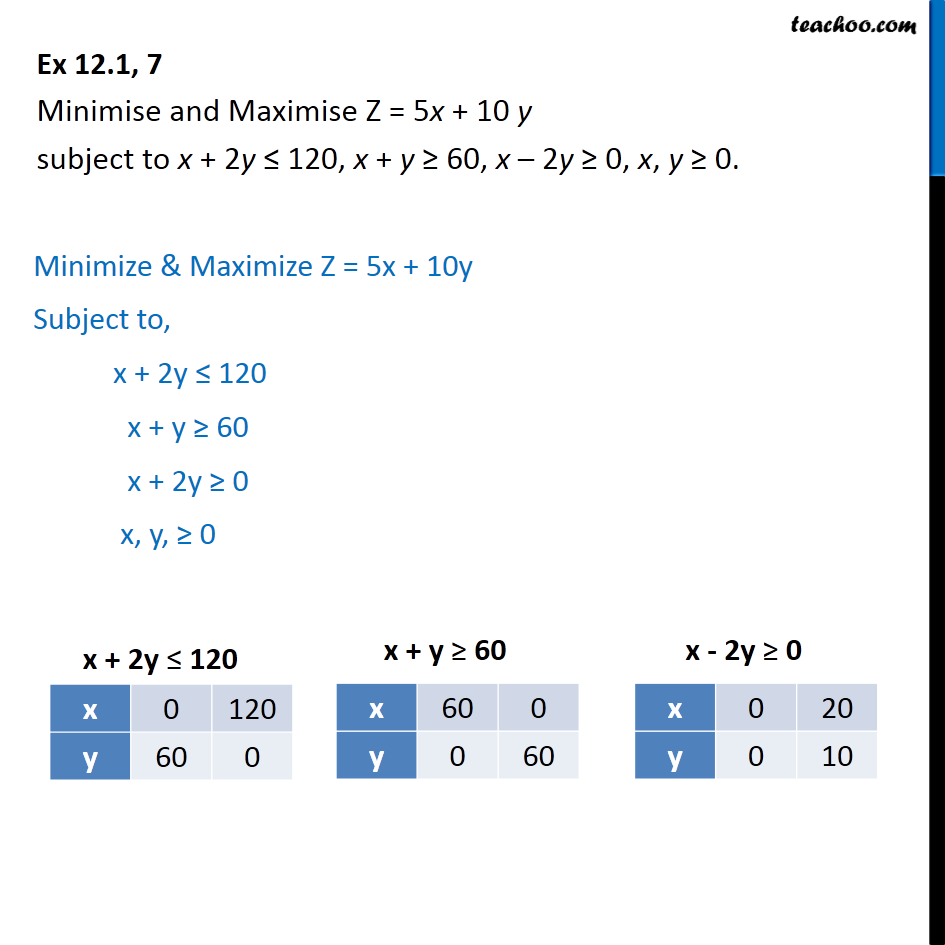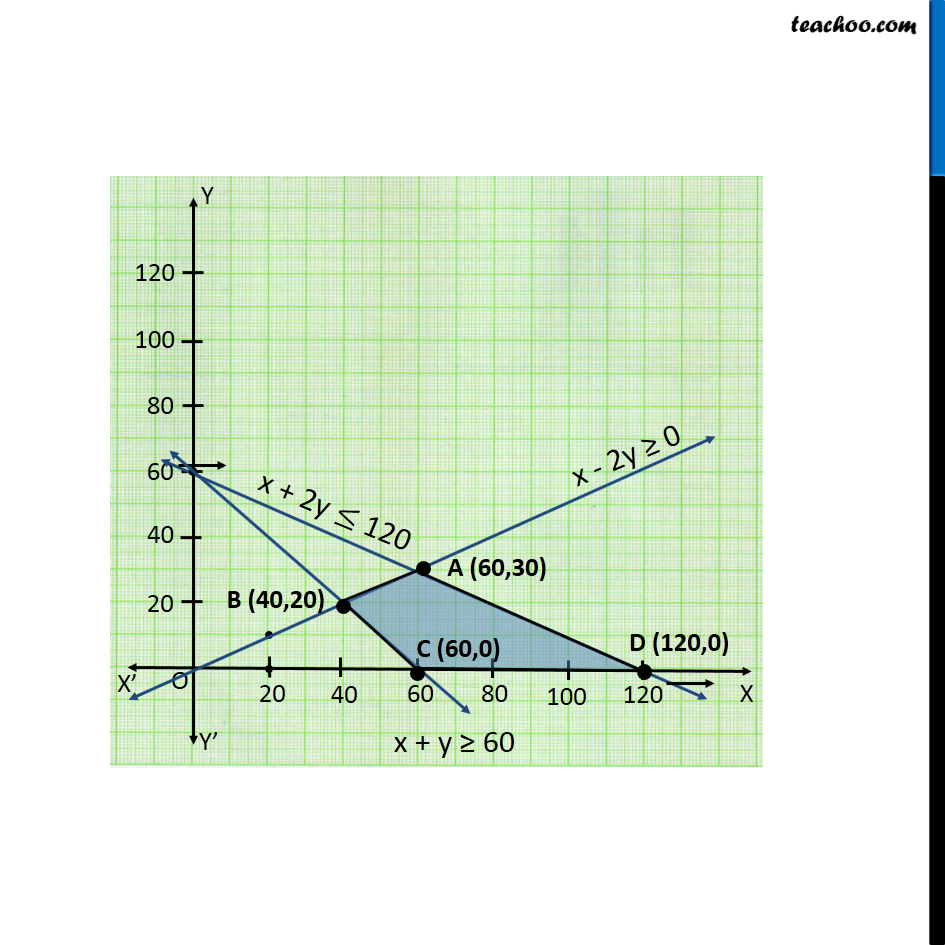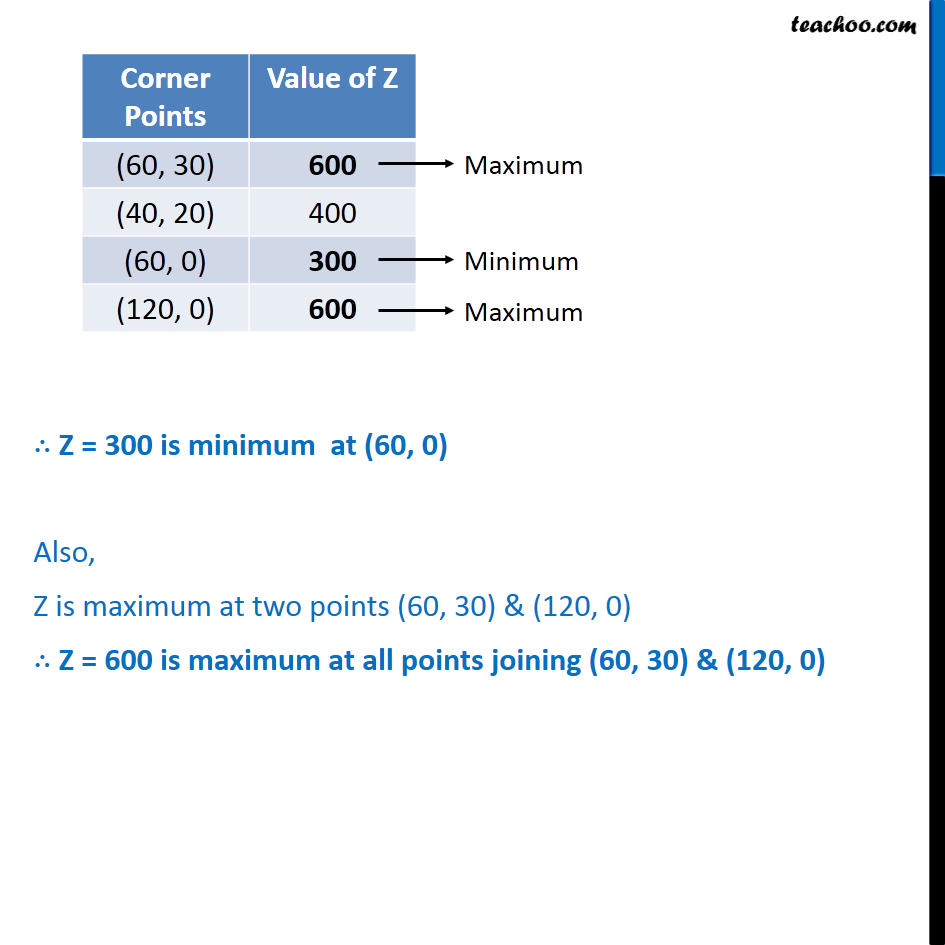1. Chapter 12 Class 12 Linear Programming
2. Serial order wise
3. Ex 12.1

Transcript

Ex 12.1, 7 Minimise and Maximise Z = 5x + 10 y subject to x + 2y 120, x + y 60, x 2y 0, x, y 0. Minimize & Maximize Z = 5x + 10y Subject to, x + 2y 120 x + y 60 x + 2y 0 x, y, 0 Z = 300 is minimum at (60, 0) Also, Z is maximum at two points (60, 30) & (120, 0) Z = 600 is maximum at all points joining (60, 30) & (120, 0)

Ex 12.1